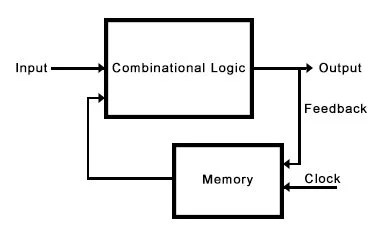View Course Path

# Sequential and Combinational logic circuits – Types of logic circuits

In any digital device, like a computer or a tablet. You will find a number of digital circuits. Digital circuits are essentially circuits that operate on the digital concept of 0s and 1s. Which means they switch on or off. So we can say that they have a unique job of switching on the application of a certain logic. And what do we mean by logic? Essentially a specific arrangement of binary codes. Consequently, these digital circuits are also known as switching circuits. There are two main types of digital logic circuits in digital electronics — combinational and sequential logic circuits.

Contents

## What are combinational logic circuits?

Combinational circuits are a basic collection of logic gates. Their outputs depend only on the current inputs. Combinational circuits are also time-independent. Along with the absence of concepts like past inputs, combinational circuits also do not require any clocks. The result of these properties is a simple circuit capable of implementing complex logic using only logic gates. An easy to understand example is a full adder.

### Block diagram of a combinational logic circuit## What are the different types of combinational logic circuits?

There are three main types of combinational logic circuits.

1. Arithmetic and logical combinational circuits – Adders, Subtractors, Multipliers, Comparators.
2. Data handling combinational circuits – Multiplexers, Demultiplexers, priority encoders, decoders.
3. Code converting combinational circuits – Binary to Gray, Gray to Binary, Binary to Excess 3, seven-segment, etc.

## What are sequential logic circuits?

Sequential circuits are a collection of memory elements. These memory elements are flip-flops. These circuits are capable of “remembering” data. Hence, a sequential circuit’s output depends on the current input, as well as past input.

Moreover, since flip-flops are present, the output of a sequential circuit also depends on the clock input. These circuits are quite complex. They are capable of implementing complex logic with memory. Add a memory element and feedback to a combinational circuit, and you get a sequential circuit.

### Block diagram of a sequential logic circuit## What are the different types of sequential logic circuits?

1. Synchronous sequential circuits– The same clock input synchronizes all the memory elements as in synchronous counters.
2. Asynchronous sequential circuits– An external clock is absent. However, the clock inputs receive pulse inputs from other sources/elements in the circuit — for example, Asynchronous counters.

## What are the differences between combinational and sequential logic circuits?

These have memory and are used to design memory storage devices

 Combinational Logic Circuits Sequential Logic Circuits Output depends only on current inputs Output depends on current, past as well as clock inputs Hence they are faster They are slower They are time-independent and don’t need clock inputs Time-dependent and thus require clocks Since there is no clock, they don’t require triggering Since a clock is present, triggering is required Made using logic gates Made using flip-flops Also, easier to design since there are no crazy feedbacks or clocks More complicated to design They can’t store anything. They have no memory. Main uses are to implement arithmetic and logical operations. The main use is for storing data and other memory applications Easier to use and handle Harder to use and handle Example: Full Adder, Multiplier etc. Example: Counters, Shift-registers

This site uses Akismet to reduce spam. Learn how your comment data is processed.

Top